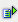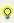Examples

The single value resp. status bits can be added to the formula with a doubleclick.

The following examples shows a simple logical AND operation with two value bits:Example Formula: X01.03 AND X02.03

This formula has the status TRUE, if bit 3 of variable 1 and bit 3 of variable 2 both have the value 1.

The value or the status of a variable can also be compared analogically:Example (X01.Value > X02.Value)

Additionally, analog values can be compared on a logical basis:Example (X01.Value> X02.Value) AND (X01.Value = X02.Value)

This is also possible with value bits and status bits.Example (X01.Value> X02.Value) AND (X01.Value = X02.Value) OR (X01.03 = X02.03)

It is also possible to compare the value with a decimal or hexadecimal value.Example Formula: (X01.Value = 111) Formula: (X01.Value = 0x6F)

If a decimal value is used, it will be displayed in hexadecimal format later. If a decimal value is entered and confirmed, the value is displayed as a hexadecimal value upon restart.Info It is not possible to use a colon or a point when entering values.